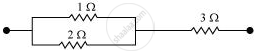Advertisement Remove all ads

# Given N Resistors Each of Resistance R, How Will You Combine Them to Get the Maximum, Minimum Effective Resistance? What is the Ratio of the Maximum to Minimum Resistance? - Physics

Given the resistances of 1 Ω, 2 Ω, 3 Ω, how will be combine them to get an equivalent resistance of (11/3) Ω

Advertisement Remove all ads

#### Solution

The resistance of the given resistors is,

R1 = 1 Ω, R2 = 2 Ω, R3 = 3 Ω2

1. Equivalent resistance, R'=11/3Omega

Consider the following combination of the resistors.Equivalent resistance of the circuit is given by,

R'=(2xx1)/(2+1)+3=2/3+3=11/3Omega

Is there an error in this question or solution?
Advertisement Remove all ads

#### APPEARS IN

NCERT Class 12 Physics Textbook
Chapter 3 Current Electricity
Q 20 | Page 129
Advertisement Remove all ads

#### Video TutorialsVIEW ALL 

Advertisement Remove all ads
Share
Notifications

View all notifications

Forgot password?